# Bokeh – Adding Widgets

• Last Updated : 29 Jul, 2021

Bokeh is a Python data visualization library for creating interactive charts & plots. It helps us in making beautiful graphs from simple plots to dashboards. Using this library, we can create javascript-generated visualization without writing any scripts.

### What is a widget?

Widgets are interactive controls that we can use with bokeh applications to make the interactive interface to visualizations. To use widgets, we can add them to the document & define their functions, or we can add them directly to the document root, added inside a layout. Two ways are allowed in bokeh to define methods for call back functionality:

• Use CustomJS callback for interactivity which works in HTML documents.
• Use bokeh server & setup event handlers with .on_change or .on_click.

These event handlers are user-defined functions in python that can be added to widgets and then called when certain actions are taken or attributes are changed in widgets. Before adding widgets to the visualization, we need to import some packages from bokeh library like:

• .io for showing the widgets & to make the output file.
• .layouts for widget box to wrap up all the widgets in it.
• .models.widgets base class for all type of interactive widgets.

And in the below example, we have used output_file() function to store the results into the file & show() to show the widgets in the browser.

### Button

It is a clickable button widget that takes three parameters in constructors:

• label: String parameter used as text label or caption for the button to display.
• icon: Optional parameter used to appear image to the left of button’s caption.
• callback: Custom javascript functions to be called when certain changes occurred like button clicking.

Here we have used button_type to specify the color like primary(blue), warning(yellow), success(green), danger(red).

## Python3

 `from` `bokeh.io ``import` `output_file, show``from` `bokeh.layouts ``import` `widgetbox``from` `bokeh.models.widgets ``import` `Button` `output_file(``"button.html"``)` `b1 ``=` `Button(label ``=` `"Back"``, button_type ``=` `"primary"``)` `show(widgetbox(b1))`

Output: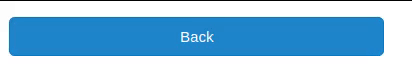This is a list of options that each contains a menu vertically. When you click one of the options from it a list of options dropdown below the main menu.

It takes three parameters:

• label: The text which is to be displayed as the title of the menu.
• button_type: To specify the button type.
• menu: To specify the menu of the options available to choose.

## Python3

 `from` `bokeh.io ``import` `output_file, show``from` `bokeh.layouts ``import` `widgetbox``from` `bokeh.models.widgets ``import` `Dropdown` `output_file(``"dropdown_menu.html"``)` `menu ``=` `[(``"Item 1"``, ``"item_1"``), (``"Item 2"``, ``"item_2"``),``        ``(``"Item 3"``, ``"item_3"``)]``dropdown_menu ``=` `Dropdown(label ``=` `"Dropdown button"``,``                         ``button_type ``=` `"warning"``,``                         ``menu ``=` `menu)` `show(widgetbox(dropdown_menu))`

Output: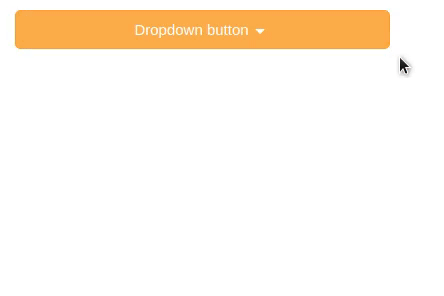### Checkbox button group

Through this widget, we can select multiple options at once.

Two parameters are given here:

• labels: To specify the name of the options to be selected.
• active: To define which of the options to be selected at once(like 0 for first options,1 for second & so on).

## Python3

 `from` `bokeh.io ``import` `output_file, show``from` `bokeh.layouts ``import` `widgetbox``from` `bokeh.models.widgets ``import` `CheckboxButtonGroup` `output_file(``"checkbox_button.html"``)` `cbg``=` `CheckboxButtonGroup(``        ``labels``=``[``"Apple"``, ``"Samsung"``, ``"Lenovo"``], active``=``[``0``, ``1``])` `show(widgetbox(cbg))`

Output: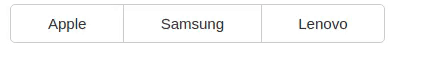### Radio button group

This widget allows selecting at most one button at a time.

Parameters:

• labels: To define name of the options.
• active: Here we can give only one value because in the radio button group only one button to select at a time.

## Python3

 `from` `bokeh.io ``import` `output_file, show``from` `bokeh.layouts ``import` `widgetbox``from` `bokeh.models.widgets ``import` `RadioButtonGroup` `output_file(``"radio_button.html"``)` `radio_button ``=` `RadioButtonGroup(``        ``labels ``=` `[``"Apple"``, ``"Mango"``, ``"Orange"``],``  ``active ``=` `0``)` `show(widgetbox(radio_button))`

Output: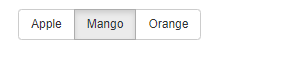### Select

It is a single selection widget which allows selecting a single value from a list of options.

The parameters used here are:

• title: To specify the title of the selection widget.
• value: Used to specify which value from the options to be selected.
• options: To specify the options to be available.

## Python3

 `from` `bokeh.io ``import` `output_file, show``from` `bokeh.layouts ``import` `widgetbox``from` `bokeh.models.widgets ``import` `Select` `output_file(``"single_select.html"``)` `select ``=` `Select(title``=``"Option:"``, value``=``"Blue"``,``                ``options``=``[``"Red"``, ``"Yellow"``, ``"Blue"``, ``"Green"``])` `show(widgetbox(select))`

Output: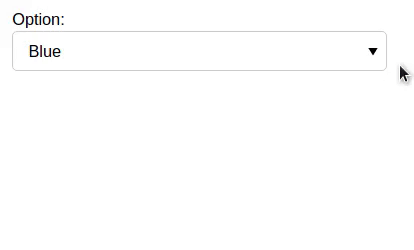### Slider

The slider has parameters like start or end value, step size, initial value, and a title.

• start: From which value the slider should start.
• end: The ending value where slider stops.
• value: In which value slider will stop.
• step: This parameter specifies the step value means the jump between the values.
• title: The title of the value in which slider stops.

## Python3

 `from` `bokeh.io ``import` `output_file, show``from` `bokeh.layouts ``import` `widgetbox``from` `bokeh.models.widgets ``import` `Slider` `output_file(``"slider.html"``)` `slider ``=` `Slider(start ``=` `0``, end ``=` `12``,``                ``value ``=` `5``, step ``=` `.``1``,``                ``title ``=` `"Average"``)` `show(widgetbox(slider))`

Output: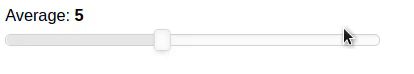### TextInput

This widget is used for collecting a line of text from the user.

• value: Initially what should be displayed before taking user input.
• title: Title of the TextInput widgets.

## Python3

 `from` `bokeh.io ``import` `output_file, show``from` `bokeh.layouts ``import` `widgetbox``from` `bokeh.models.widgets ``import` `TextInput` `output_file(``"text_input.html"``)` `text ``=` `TextInput(value ``=` `"",``                ``title ``=` `"Label:"``)` `show(widgetbox(text))`

Output: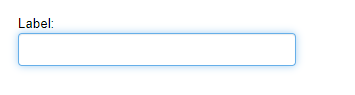### Paragraph

Used to display a block of text.

• text: Text which is to be displayed.
• width: height: To specify the width and height of the paragraph widget.

## Python3

 `from` `bokeh.io ``import` `output_file, show``from` `bokeh.layouts ``import` `widgetbox``from` `bokeh.models.widgets ``import` `Paragraph` `output_file(``"para.html"``)` `para ``=` `Paragraph(text ``=` `"""Encryption is the process of converting``normal message (plaintext) into meaningless message (Cipher text).``Whereas Decryption is the process of converting meaningless message``(Cipher text) into its original form (Plaintext)."""``,``width ``=` `250``, height ``=` `80``)` `show(widgetbox(para))`

Output: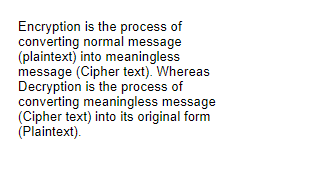### TextareaInput

This is used to store multiple lines of text from users.

• value: The default value of the Textarea widget.
• rows: Number of rows to be given as a space for text input.
• title: To specify the title of the Textarea widget.

## Python3

 `from` `bokeh.io ``import` `show``from` `bokeh.models ``import` `TextAreaInput` `text_area ``=` `TextAreaInput(value ``=` `"Write here"``,``                          ``rows ``=` `6``, title ``=` `"Label:"``)``show(text_area)`

Output: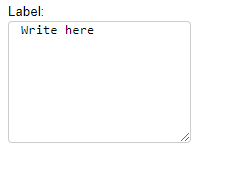This hides the entered text input which is used for password input.

• placeholder: A short hint about the data which is to be entered in input area and when the user input value this will be removed.

## Python3

 `from` `bokeh.io ``import` `show``from` `bokeh.models ``import` `PasswordInput` `password ``=` `PasswordInput(placeholder ``=` `"Enter password..."``)``show(password)`

Output: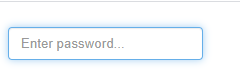### Pretext:

Used to display pre-formatted text.

• text: Text which is to be displayed.
• width, height: To specify the width and height of widget.

## Python3

 `from` `bokeh.io ``import` `show``from` `bokeh.models ``import` `PreText` `pretext ``=` `PreText(text``=``"""Encryption is the process of converting``normal message (plaintext) into meaningless message (Cipher text).``Whereas Decryption is the process of converting meaningless message``(Cipher text) into its original form (Plaintext)"""``,``width ``=` `500``, height ``=` `120``)` `show(pretext)`

Output: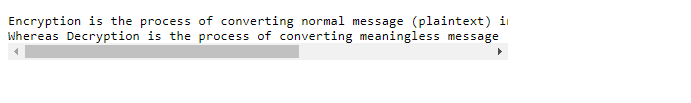It is a collection of radio boxes.

• labels: To specify the value of the options available to select.
• active: Default value which is shown as selected before choosing any option.

## Python3

 `from` `bokeh.io ``import` `output_file, show``from` `bokeh.layouts ``import` `widgetbox``from` `bokeh.models.widgets ``import` `RadioGroup` `output_file(``"radio_group.html"``)` `radio_g ``=` `RadioGroup(``        ``labels ``=` `[``"AI"``, ``"ML"``, ``"Deep Learning"``],``  ``active ``=` `1``)` `show(widgetbox(radio_g))`

Output: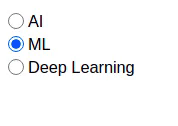### Div:

It is a small section or container in which various styling of that section can be done.

• text: Content which is to be displayed.
• width, height: To specify the width and height of this widget.

## Python3

 `from` `bokeh.io ``import` `output_file, show``from` `bokeh.layouts ``import` `widgetbox``from` `bokeh.models.widgets ``import` `Div` `output_file(``"div.html"``)` `div ``=` `Div(text``=``"""``Python is high level programming language.``Its easy to learn because of its syntax."""``,``width ``=` `250``, height ``=` `100``)` `show(widgetbox(div))`

Output: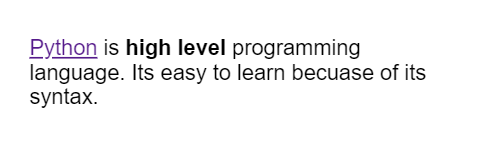### Toggle

Used to display the checked/unchecked state of the button or to change the setting between these two states.

• label: Text to be displayed as the title of the button.
• button_type: To specify the color of the button.
• width, height: To specify the width and height of the button.

## Python3

 `from` `bokeh.io ``import` `output_file, show``from` `bokeh.models.widgets ``import` `Toggle` `output_file(``"toggle.html"``)` `toggle ``=` `Toggle(label ``=` `"Switch"``,``                ``button_type ``=` `"success"``,``                ``width ``=` `250``, height ``=` `100``)` `show(toggle)`

Output: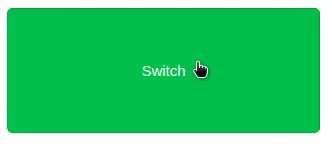### FileInput:

This allows the user to choose a file and store its information.

## Python3

 `from` `bokeh.io ``import` `show``from` `bokeh.models.widgets ``import` `FileInput` `file` `=` `FileInput()` `show(``file``)`

Output: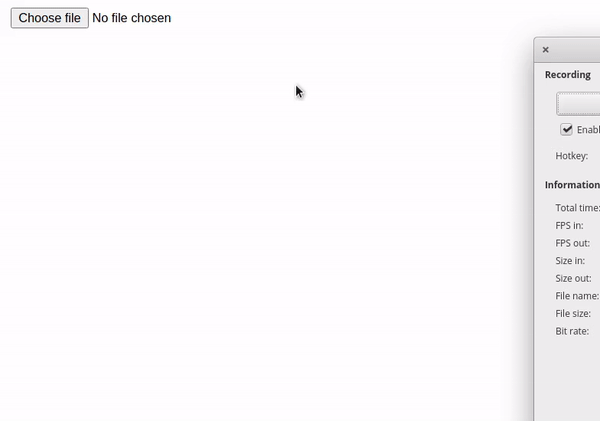### Spinner:

It provides a quick way to select one value from a set. In this widget, we have used different libraries like NumPy, etc.

• np.random.rand: To generate random numbers.
• figure: To make a figure for scatter plot.

In spinner constructor different parameters are given like:

• title: For giving title to spinner.
• low, high: To specify the lowest and highest possible value allowed for increasing the size of points in dropdown menu.
• step: This parameter specifies the step value means the jump between the values.
• value: Default value for menu.
• width: To define the width of the spinner.

And here we have linked two bokeh model properties using custom js callbacks(js_link) to update the properties of the model whenever certain actions are done.

## Python3

 `import` `numpy as np` `from` `bokeh.io ``import` `show``from` `bokeh.layouts ``import` `column, row``from` `bokeh.models ``import` `Spinner``from` `bokeh.plotting ``import` `figure` `x ``=` `np.random.rand(``10``)``y ``=` `np.random.rand(``10``)` `a ``=` `figure(x_range``=``(``0``, ``1``), y_range``=``(``0``, ``1``))``points ``=` `a.scatter(x ``=` `x, y ``=` `y, size ``=` `4``)` `spinner ``=` `Spinner(title``=``"Glyph size"``, low ``=` `1``,``                  ``high ``=` `40``, step ``=` `0.5``, value ``=` `4``, width ``=` `80``)``spinner.js_link(``'value'``, points.glyph, ``'size'``)` `show(row(column(spinner, width ``=` `100``), a))`

Output: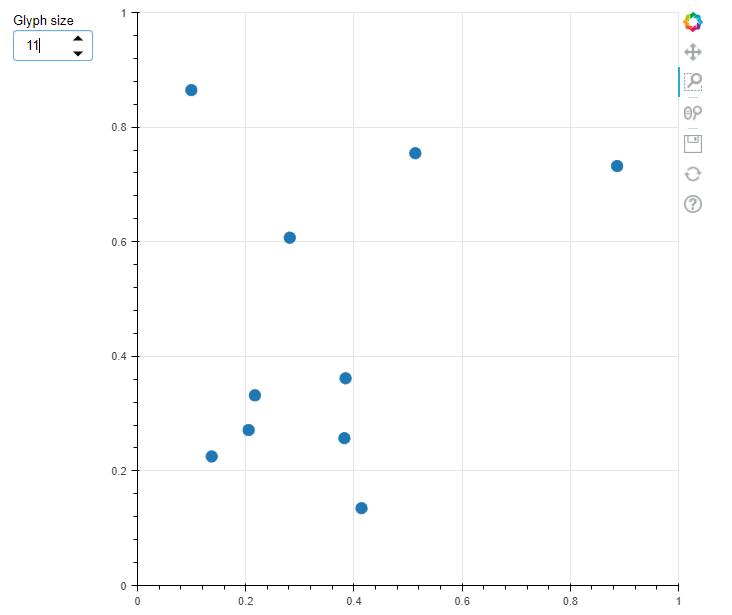### Tabs:

This widget allows multiple plots to be displayed in configurable panels. The layout of this widget consists of two bokeh models i.e. Tab() and Panel().

Here we have used figure() to make a figure for plotting and Panel() which is a container having title and control.

• circle(): To make circle plot.
• line(): To make a line plot.

## Python3

 `from` `bokeh.io ``import` `show``from` `bokeh.models ``import` `Panel, Tabs``from` `bokeh.plotting ``import` `figure` `p1 ``=` `figure(plot_width``=``350``, plot_height``=``300``)``p1.circle([``1``, ``2``, ``3``, ``4``, ``5``], [``6``, ``7``, ``2``, ``4``, ``5``],``          ``size ``=` `20``, color ``=` `"red"``, alpha ``=` `0.5``)` `tab1 ``=` `Panel(child``=``p1, closable ``=` `True``,``             ``title ``=` `"circle"``)` `p2 ``=` `figure(plot_width ``=` `350``, plot_height ``=` `300``)``p2.line([``1``, ``2``, ``3``, ``4``, ``5``], [``6``, ``7``, ``2``, ``4``, ``5``],``        ``line_width ``=` `3``, color ``=` `"blue"``, alpha ``=` `0.5``)``tab2 ``=` `Panel(child ``=` `p2, closable ``=` `True``, title ``=` `"line"``)` `show(Tabs(tabs ``=` `[tab1, tab2]))`

Output: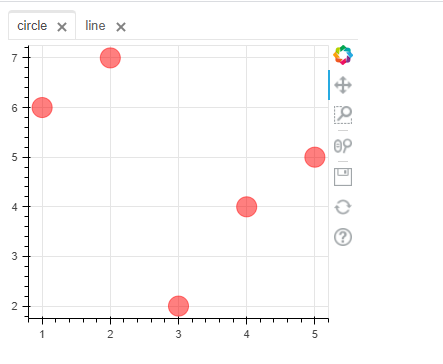### DateRangeSlider:

It is used to select the date range with a slider.

Parameters used are:

• value: The default date range value which is to be displayed.
• start: To specify the starting value from where the slider should start.
• end: To specify the ending value from where the slider should stop.

## Python3

 `from` `datetime ``import` `date``from` `bokeh.io ``import` `show``from` `bokeh.models ``import` `CustomJS, DateRangeSlider` `date_range``=` `DateRangeSlider(value ``=` `(date(``2020``, ``1``, ``9``),``                                     ``date(``2021``, ``1``, ``10``)),``                            ``start ``=` `date(``2019``, ``8``, ``12``),``                            ``end ``=` `date(``2021``, ``6``, ``9``))` `show(date_range)`

Output: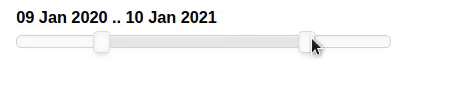### DatePicker:

It is a calendar-based date selection widget.

• title: Text which is to be shown as the title of the widget.
• value: Default value to be displayed when options are not selected.
• min_date: Minimum date means from which month & date the calendar will start.
• max_date: Maximum date means from which month & date the calendar will be ended.

## Python3

 `from` `bokeh.io ``import` `show``from` `bokeh.models ``import` `CustomJS, DatePicker` `dp ``=` `DatePicker(title ``=` `'Select date'``, value ``=` `"2021-06-09"``,``                ``min_date ``=` `"2020-10-01"``, max_date ``=` `"2021-12-31"``)``show(dp)`

Output: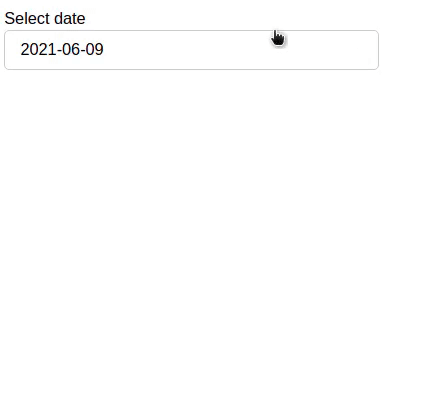### DataTable:

It is based on a slick grid which takes external components as data sources. And any plots which contain this data will automatically be linked with the plots and table.

Here we have made a dictionary for columns value and then mapped the list of data which is in form of a dictionary with a column using ColumnDataSource() function after that we make the layout of the column using TableColumn() having arguments as-

• field: Column contains what values.
• title: Title of column.
• formatter: To make the format of date column as date.

DataTable constructor contains:

• source: To define the mapped data with column as source.
• Column: To specify the column values.
• width, height: To define the width, height of the table.

## Python3

 `from` `datetime ``import` `date``from` `random ``import` `randint` `from` `bokeh.io ``import` `show``from` `bokeh.models ``import` `ColumnDataSource, DataTable, DateFormatter, TableColumn` `data ``=` `dict``(``        ``dates``=``[date(``2021``, ``5``, i``+``1``) ``for` `i ``in` `range``(``10``)],``        ``downloads``=``[randint(``0``, ``130``) ``for` `i ``in` `range``(``10``)],``    ``)``source ``=` `ColumnDataSource(data)` `columns ``=` `[``        ``TableColumn(field ``=` `"dates"``, title ``=` `"Date"``,``                    ``formatter ``=` `DateFormatter()),``        ``TableColumn(field ``=` `"downloads"``, title ``=` `"Downloads"``),``    ``]``data_table ``=` `DataTable(source ``=` `source, columns ``=` `columns,``                       ``width ``=` `400``, height ``=` `280``)` `show(data_table)`

Output: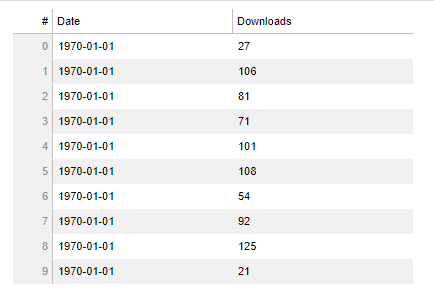### ColorPicker:

This provides the user to select RGB color value.

• Figure: To make a figure for plotting.
• plot.line: Used to make a line plot.

## Python3

 `from` `bokeh.io ``import` `show``from` `bokeh.layouts ``import` `column``from` `bokeh.models ``import` `ColorPicker``from` `bokeh.plotting ``import` `Figure` `plot ``=` `Figure(x_range``=``(``0``, ``1``), y_range``=``(``0``, ``1``),``              ``plot_width``=``350``, plot_height``=``400``)``line ``=` `plot.line(x``=``(``0``,``1``), y``=``(``0``,``1``), color``=``"green"``,``                 ``line_width``=``4``)` `picker ``=` `ColorPicker(title``=``"Line Color"``)``picker.js_link(``'color'``, line.glyph, ``'line_color'``)` `show(column(plot, picker))`

Output: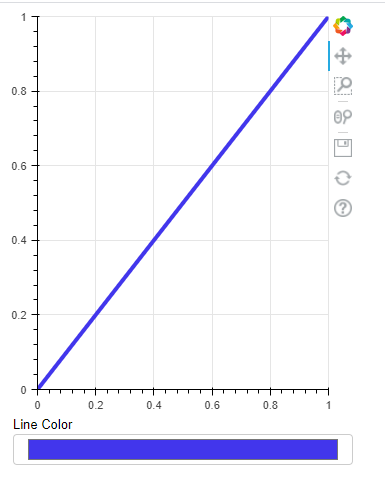My Personal Notes arrow_drop_up